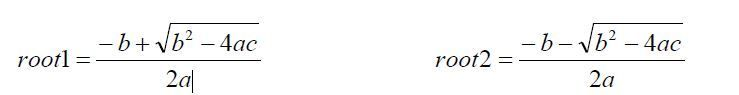Posted by Samath Last Updated: March 02, 20154521

The roots of a quadratic equation ax2+bx+c are given by the quadratic formulaWrite a function quadratic in python which returns the greater of the two roots. However, the root is returned when the discriminant (i.e. b2-4ac) is positive when it is negative print a message that there are no real roots.

Code:

```import math